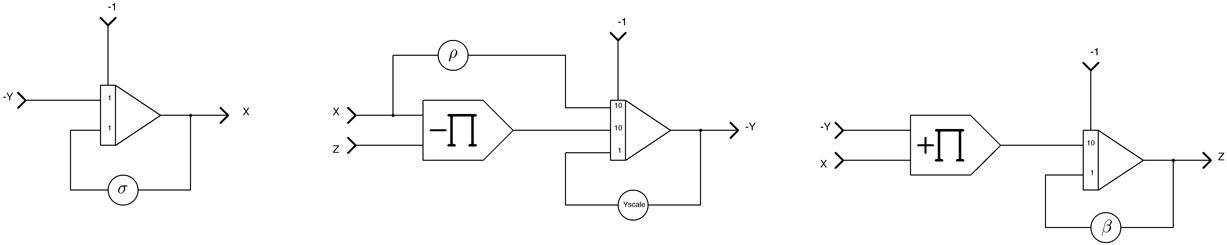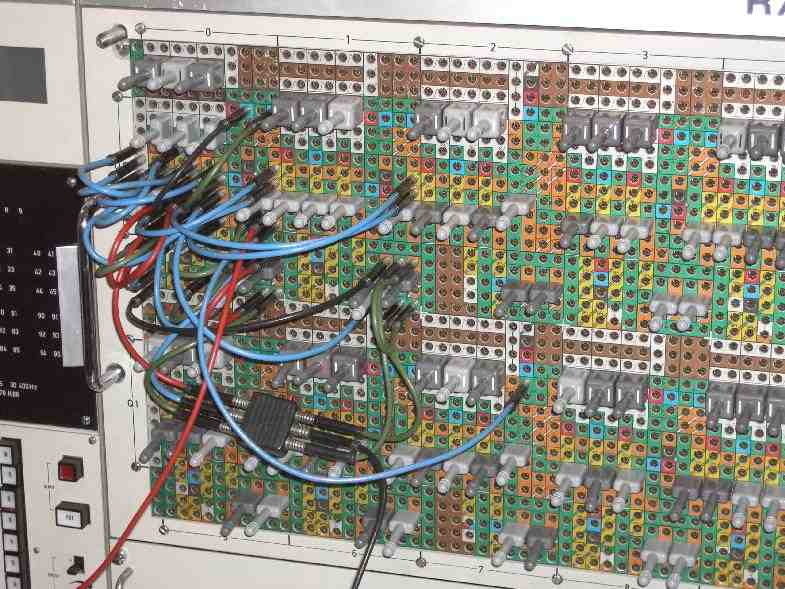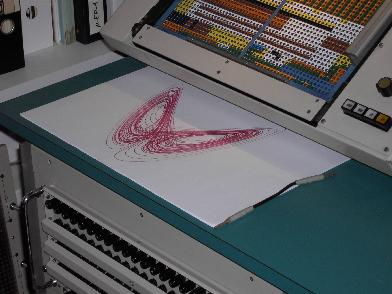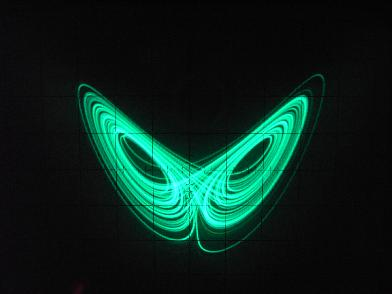# Lorenz Attractor

 Nearly everybody has seen pictures of the so called Lorenz attractor, discovered by Edward N. Lorenz. This attractor is a plot of the phase state of a system of the three coupled differential equations shown on the right (stolen from the Wikipedia page linked to above). Normally this system of equations is solved numerically employing standard digital techniques but being a system of differential equations it is natural to seek for a solution based on an electronic analog computer (this has been shown by Paul Horowitz who developed a simple circuit using only three operational amplifiers and two analog multipliers which can be seen here).These three equations can be mechanized as follows in form of three analog computer circuits which have to be interconnected to get a working program - in this case for the Telefunken RA 770 analog computer (circuit drawing by Arno Jacobs):Implementing these three subcircuits on a Telefunken RA 770 analog computer yields the setup shown below:One of the most wonderful characteristics of an electronic analog computer is its ability to change time scaled of a computation easily. All that is necessary is to change the size of the feedback capacitors used in the integrators, so it is possible to either plot the Lorenz attractor using a pen plotter (below left) or to display the evolving Lorenz attractor on an oscilloscope which gives a rather tantalizing view (below right). The parameters used were a = 0.357, b = 0.1 and c = 0.374.03-JAN-2010, ulmann@analogmuseum.org